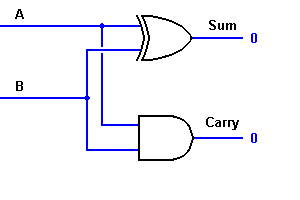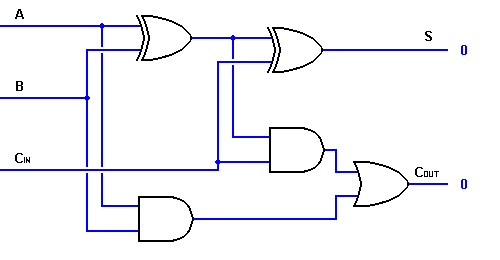## Audio

[Audio][slideshow]

Before going into this subject, it is very important to know about Boolean Logic and Logic Gates.

TAKE A LOOK : BOOLEAN LOGIC

TAKE A LOOK : LOGIC GATES

TAKE A LOOK : FLIP FLOPS

With the help of half adder, we can design circuits that are capable of performing simple addition with the help of logic gates.

Let us first take a look at the addition of single bits.

0+0 = 0

0+1 = 1

1+0 = 1

1+1 = 10

These are the least possible single-bit combinations. But the result for 1+1 is 10. Though this problem can be solved with the help of an EXOR Gate, if you do care about the output, the sum result must be re-written as a 2-bit output.

Thus the above equations can be written as

0+0 = 00

0+1 = 01

1+0 = 01

1+1 = 10

Here the output ‘1’of ‘10’ becomes the carry-out. The result is shown in a truth-table below. ‘SUM’ is the normal output and ‘CARRY’ is the carry-out.

INPUTS OUTPUTS

A B SUM CARRY

0 0 0 0

0 1 1 0

1 0 1 0

1 1 0 1

From the equation it is clear that this 1-bit adder can be easily implemented with the help of EXOR Gate for the output ‘SUM’ and an AND Gate for the carry. Take a look at the implementation below.For complex addition, there may be cases when you have to add two 8-bit bytes together. This can be done only with the help of full-adder logic.

This type of adder is a little more difficult to implement than a half-adder. The main difference between a half-adder and a full-adder is that the full-adder has three inputs and two outputs. The first two inputs are A and B and the third input is an input carry designated as CIN. When a full adder logic is designed we will be able to string eight of them together to create a byte-wide adder and cascade the carry bit from one adder to the next.

The output carry is designated as COUT and the normal output is designated as S. Take a look at the truth-table.

INPUTS OUTPUTS

A B CIN COUT S

0 0 0 0 0

0 0 1 0 1

0 1 0 0 1

0 1 1 1 0

1 0 0 0 1

1 0 1 1 0

1 1 0 1 0

1 1 1 1 1

From the above truth-table, the full adder logic can be implemented. We can see that the output S is an EXOR between the input A and the half-adder SUM output with B and CIN inputs. We must also note that the COUT will only be true if any of the two inputs out of the three are HIGH.

Thus, we can implement a full adder circuit with the help of two half adder circuits. The first will half adder will be used to add A and B to produce a partial Sum. The second half adder logic can be used to add CIN to the Sum produced by the first half adder to get the final S output. If any of the half adder logic produces a carry, there will be an output carry. Thus, COUT will be an OR function of the half-adder Carry outputs. Take a look at the implementation of the full adder circuit shown below.Though the implementation of larger logic diagrams is possible with the above full adder logic a simpler symbol is mostly used to represent the operation. Given below is a simpler schematic representation of a one-bit full adder.